[BACK]
 Computers, Materials & ContinuaDOI:10.32604/cmc.2022.029134Article

Deep CNN Model for Multimodal Medical Image Denoising

1Department Electronics and Electrical Communications, Faculty of Electronic Engineering, Menoufia University, Menouf, 32952, Egypt
2Security Engineering Laboratory, Department of Computer Science, Prince Sultan University, Riyadh, 11586, Saudi Arabia
3Alexandria Higher Institute of Engineering & Technology (AIET), Alexandria, Egypt
4Department of Information Technology, College of Computer and Information Sciences, Princess Nourah bint Abdulrahman University, Riyadh, 11671, Saudi Arabia
5Department of Computer Sciences, College of Computer and Information Sciences, Princess Nourah bint Abdulrahman University, Riyadh, Saudi Arabia
*Corresponding Author: Amel A. Alhussan. Email: aaalhussan@pnu.edu.sa
Received: 25 February 2022; Accepted: 30 March 2022

Abstract: In the literature, numerous techniques have been employed to decrease noise in medical image modalities, including X-Ray (XR), Ultrasonic (Us), Computed Tomography (CT), Magnetic Resonance Imaging (MRI), and Positron Emission Tomography (PET). These techniques are organized into two main classes: the Multiple Image (MI) and the Single Image (SI) techniques. In the MI techniques, images usually obtained for the same area scanned from different points of view are used. A single image is used in the entire procedure in the SI techniques. SI denoising techniques can be carried out both in a transform or spatial domain. This paper is concerned with single-image noise reduction techniques because we deal with single medical images. The most well-known spatial domain noise reduction techniques, including Gaussian filter, Kuan filter, Frost filter, Lee filter, Gabor filter, Median filter, Homomorphic filter, Speckle reducing anisotropic diffusion (SRAD), Nonlocal-Means (NL-Means), and Total Variation (TV), are studied. Also, the transform domain noise reduction techniques, including wavelet-based and Curvelet-based techniques, and some hybridization techniques are investigated. Finally, a deep (Convolutional Neural Network) CNN-based denoising model is proposed to eliminate Gaussian and Speckle noises in different medical image modalities. This model utilizes the Batch Normalization (BN) and the ReLU as a basic structure. As a result, it attained a considerable improvement over the traditional techniques. The previously mentioned techniques are evaluated and compared by calculating qualitative visual inspection and quantitative parameters like Peak Signal-to-Noise Ratio (PSNR), Correlation Coefficient (Cr), and system complexity to determine the optimum denoising algorithm to be applied universally. Based on the quality metrics, it is demonstrated that the proposed deep CNN-based denoising model is efficient and has superior denoising performance over the traditional denoising techniques.

Keywords: Image enhancement; medical imaging; speckle noise; Gaussian noise; denoising filters; CNN denoising

1  Introduction

Medical scans are great tools that help specialists to identify the different abnormalities in the body organs. These scans can detect, diagnose, and treat different diseases. The main used medical scans are Us, XR, CT, PET, and MRI . Unfortunately, Us images suffer from the speckle noise , resulting from destructive interference between the reflected echoes. X-ray, CT, PET, and MRI imaging systems are sometimes pervaded by Gaussian noise , which is a statistical artifact. It arises mainly from sensor noise, heat, propagation, or circuit noise. In the literature work [8,9], frame and traditional models have been studied to enhance the quality of different image modalities. Noise removal techniques are a preprocessing stage commonly used in computer vision, such as classification, recording, segmentation, and reconstruction image. Therefore, traditional techniques are used to remove noise from medical images .

As shown in Fig. 1, we have selected some well-known noise reduction techniques. The first group is the spatial domain techniques, including the Gaussian filter , which is simple to implement but loses a few image details. Gabor filter  cannot remove image noise, especially at high noise levels. Lee filter [13,14] is a well-known adaptive local filter that takes the mean of neighborhood pixels for denoising and preserves the edges and other image details, but it is disliked because it eliminates the noise nearest area of edges. Frost filter  uses an exponential kernel. It reduces the image noise, but it smooths the image and takes more computation time. Kuan filter  is better than the Lee filter in eliminating noise and preserving the edges nearest the boundaries of the images, but its parameters need to be determined properly.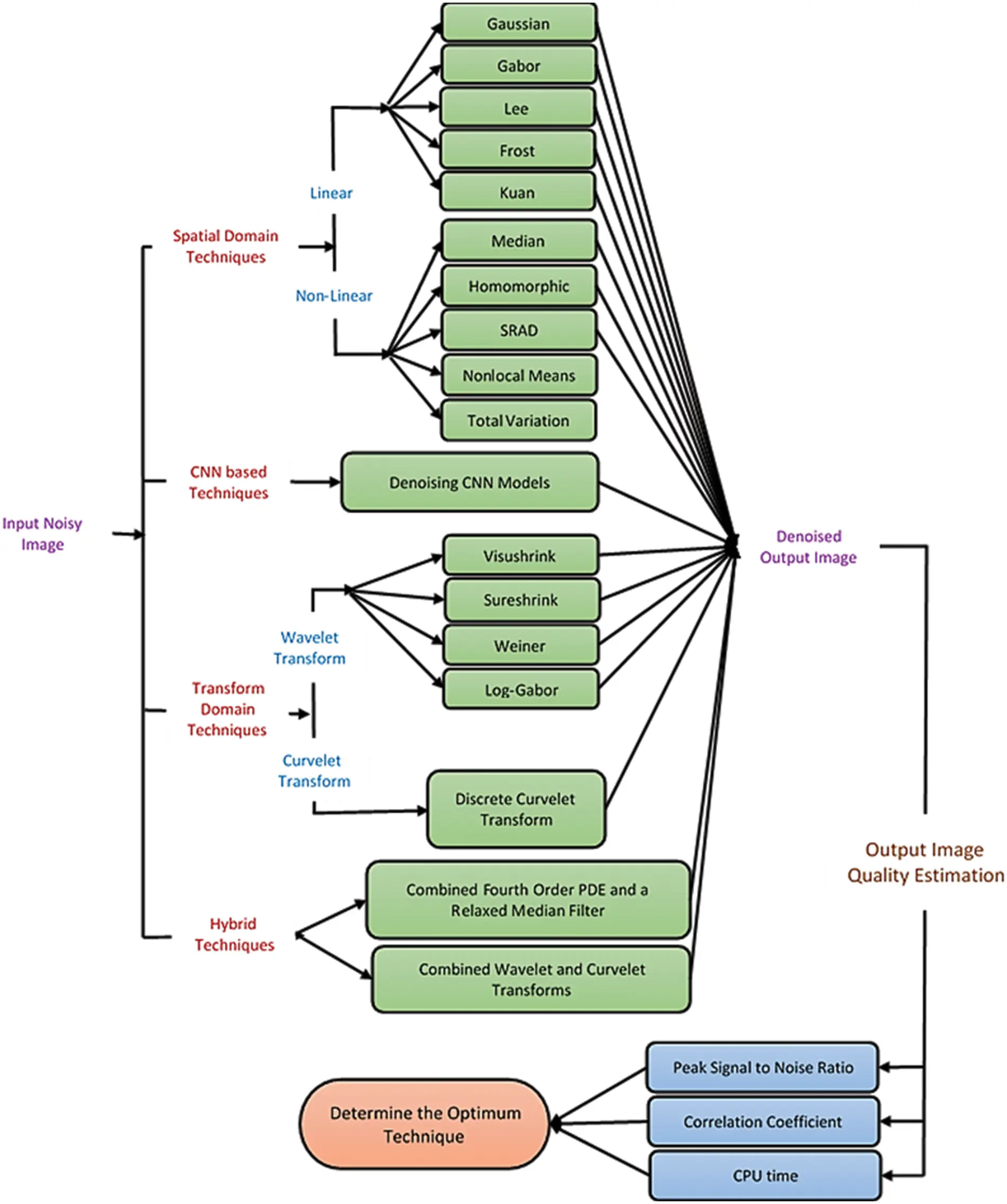Figure 1: The main goal and motivation of the work

Median filter [16,17] is a non-linear filter, whose method is based on the median pixel value of its nearby neighbors, sustains the edge. However, in the case of speckle noise, it results in blurred images or reduced information in the edge . Homomorphic filter [19,20] gradually reduces noise and improves the image contrast. SRAD [21,22] can effectively reduce noise and preserve image edges, but its performance degrades at high noise variances. The restored pixel value NL-Means filter  depends on the weighted average of surrounding pixels. It gives good denoising performance. The TV technique  is based on the principle that images with excessive features have huge total variation, So, it eliminates the noise while sustaining important features such as edges. The other group is the transform domain noise reduction techniques, including wavelet-based  techniques and curvelet-based techniques. Weiner filter  based on wavelet transform is efficient to remove noise from the image, smooth the image, and sustain the edges but partly removes noise from the bright region and reduces the brightness of the image.

The Log-Gabor filter  is particularly useful in image denoising because it can better capture the features of the image. On the other hand, curvelet-based techniques are most acceptable for image denoising as they present the image edges . There are some hybridization techniques as the combined fourth-order PDE and a relaxed median filter algorithm (Hybrid1)  and the combined Wavelet and Curvelet Transform Algorithm (Hybrid2) . Finally, a proposed CNN-based denoising model is proposed to eliminate Speckle and Gaussian noise in different modalities of medical images. The denoising CNN achieved a significant performance improvement over conventional techniques in image denoising using convolution, BN, and ReLU as a basic structure. We evaluated and compared these techniques by calculating qualitative visual inspection and quantitative parameters like PSNR, Cr, and system complexity . As a result, we can suggest the best technique that suits each imaging modality and the features of the denoised medical images. Therefore, we can determine an optimum denoising algorithm to be applied universally.

The major impacts of this research work are:

•   Presenting a comparative analysis of traditional medical image denoising techniques and the proposed CNN-based denoising model for multi-modal images.

•   It is found that the denoising CNN model has a superior performance in contrast to other denoising models.

•   The denoising CNN can easily handle different medical images with different characteristics and different noise types. Therefore, the CNN-based denoising model can improve performance more than other models for various noise levels.

The rest of this paper is arranged as follows. Section 2 reviews the medical image noise reduction techniques. Section 3 illustrates the suggested CNN-based noise reduction model. Section 4 analyses the outcomes and discusses the obtained various results. Section 5 shows the concluding remarks.

2  Medical Image Noise Reduction Techniques

In this paper, we are concerned with single image noise reduction techniques. Single-image noise reduction techniques can be carried out in the spatial or transform domains [5,10].

2.1 Spatial Domain-Based Noise Reduction Techniques

2.1.1 Linear Filtering Techniques

•   Gaussian Filter

It has an impulse response which is a Gaussian function. It can be represented as :

f(x,y)=14πσ2e(x2+y2)/2σ2(1)

•   Gabor Filter

The impulse response of the Gabor filter  is specified by a sinusoidal function multiplied by a Gaussian kernel. It includes real and imaginary parts illustrating perpendicular directions. These two parts may be arranged into a complex number or employed separately.

The complex form is given by:

g(x,yλ,θ,σ,γ)=exp(x2+γ2y22σ2)exp(i(2πxλ+ψ))(2)

The real is:

g(x,yλ,θ,σ,γ)=exp(x2+γ2y22σ2)cos(2πxλ+ψ)(3)

The imaginary is:

g(x,yλ,θ,σ,γ)=exp(x2+γ2y22σ2)sin(2πxλ+ψ)(4)

where x=xcosθ+ysinθ and y=xsinθ+ycosθ

•   Lee Filter

It is based on the idea that the filtering will be carried out if the variance in a specific region is low or uniform [13,14]. Apart from that, filtering will not be implemented. Let I be an image pixel corrupted by noise n; the lee filter has the general form:

I^=I¯n+[InI¯n]wl(5)

where wl is the weighting function for Lee filter ranging between 0 for uniform regions and 1 for non-uniform regions, I¯n is the mean of pixels in a moving window, In is the noisy image, and I^ is the output image.

•   Frost Filter

It is an adaptive filter suitable for noise reduction [15,16]. It acts as a mean filter in smooth or uniform regions and a high-pass filter in non-uniform regions. The Frost filter is formed as:

I^=In1M1+In2M2++InnMnM1+M2++Mn(6)

where M is the exponential weighting factor.

•   Kuan Filter

It is similar to the Lee filter but with various weighting functions [14,15]. It is computed in the same way as the Lee filter:

I^=I¯n[InI¯n]wk(7)

The weighting function of the Kuan filter is defined as:

wk=σ2σ2+(I¯n2+σ2)/L(8)

2.1.2 Non-Linear Filtering Techniques

•   Median filter

Median filtering is implemented by first arranging all pixels from the neighborhood into a numeral arrangement, and the median of these values is computed, and then the filtered pixels are replaced with the computed median .

•   Homomorphic Filter

Generally, an image can be considered as a 2-D function.

I(x,y)=R(x,y).L(x,y)(9)

The product of illumination (L) and reflectance (R) results in intensity (I). Since illumination and reflectance are multiplicative components, they are turned into additive by applying the logarithm on the image intensity . These components are separated linearly in the frequency domain. So, noise can be minimized by filtering in the log domain .

•   Speckle Reducing Anisotropic Diffusion (SRAD)

In fact, it is shown that the SRAD [21,22] can be related directly to the Lee and Frost window-based filters. It reduces the noise via solving a partial differential equation (PDE) and identifying the edges. Given an image I0(x,y) over the 2-D coordinate grid Ω, the output image I(x,yt) is evolved according to the following PDE:

I(x,yt)={I(x,yt)t=div[c(q)I(x,yt)]I(x,y0)=I0(x,y),I(x,yt)n|Ω=0(10)

where Ω denotes the border of Ω, n is the outer normal to the Ω and c(q) is the diffusion coefficient.

•   Non-Local Means Filtering

For the non-local means filter , the estimated value NL[v](i) for a pixel i, is computed as a weighted average of all the pixels in the image for a given discrete noisy image v={v(i)|iI}.

NL[v](i)=jIw(i,j)v(j)(11)

The filter weights can be represented as:

w(i,j)=1z(i)ev(v(Ni)v(Nj))2,a2(12)

where z(i) is the normalizing constant.

•   Total Variation Minimization Algorithm

It was presented in . According to this principle, reducing the total variation of the noisy image makes it a close match to the ground truth image and removes the unwanted detail while preserving important details such as edges. It’s mathematical given as follows:

TVFλ(v)=argminuTV(u)+λ|v(x)u(x)|2dx(13)

where TV(u) denotes the total variation of u and λ is a given Lagrange multiplier. v(x) and u(x) are the original and noisy images.

2.2 Transform Domain-Based Noise Reduction Techniques

2.2.1 Wavelet Domain

•   Visu-Shrink

Visu-shrink makes use of the global thresholding scheme. It adopts a hard threshold value T that is proportional to the standard noise deviation σn as follows :

T=σn2log10m(14)

where m is the image size.

•   Sure-Shrink

Sure-shrink denoises an image by applying a soft threshold on the detail coefficients . The objective of Sure shrink is to retain MSE as minimum as possible; it is defined as:

MSE=1n2x,y=1n(I^(x,y)I(x,y))2(15)

•   Wiener Filter

Wiener filter belongs to a category of optimal linear filters. It gives a linear estimate of the image from its noisy version. Therefore, this filter needs information about the noise spectrum and the noise-free image .

•   Log Gabor Filter

The transfer function of the Log-Gabor is given as :

G(f)=elog(f/f0)2/2log(σ/f0)2(16)

where f0 is the center frequency of the filter and σ/f0 is the standard deviation of the Gaussian kernel.

The denoising procedure of the log-Gabor filter  is simple to implement and has the following steps:

•   Multi-scale decomposition of the noisy image.

•   Log-Gabor filtering of all sub-bands except for the approximation band.

•   Soft thresholding of the filtered sub-bands.

•   Inverse Discrete Wavelet Transform (DWT).

2.2.2 Curvelet Domain

•   Discrete Curvelet Transform Algorithm

One of the weaknesses of the wavelet transform is that it is poor at extracting features from curves and edges of images, unlike curvelet transforms. The curvelet transform  is the development of the Ridgelet transform to identify arched borders efficiently.

2.3 Hybrid Noise Reduction Techniques

•   Combined Fourth-Order PDE and a Relaxed Median Filter Algorithm (Hybrid1)

The hybrid filter combines the advantages of a fourth-order PDE and a comfortable intermediate filter  and is called the hybrid1 algorithm. Hybrid1 preserves subtle features, curved structures, sharp angles, and edges without compromising the features of the spatial neighborhood.

•   Combined Wavelet and Curvelet Transform Algorithm (Hybrid2)

It is unsuitable for representing the high-level dimension singularities. On the other hand, the curvelet transform is used because it is robust when dealing with image edges, lines, and curves. So, combining both wavelet and curvelet transforms is superior to dealing with noisy images, unlike wavelet only or curvelet only.

3  Proposed Deep CNN-Based Medical Image Denoising Model

In the field of removing noise from images, deep learning structures have been presented for their high quality compared to traditional algorithms. This paper proposes deep learning models to reduce noise from medical images. Deep learning is characterized by its high efficiency; more data is used in the training phase. The proposed model for noise reduction consists of deep residual layers with BN. The remaining layers are distinguished by their ability to differentiate between real features and noise-generated features, and BN was used to achieve stability and speed up the training process. Our proposed model is considered a modification to the model in  research to make it suitable for reducing noise from medical images. The medical images differ from the rest of the images in the ambiguity of the features and the difficulty of perceiving them, like other digital images. The modification was done by adding deep residual layers and merging them with BN to improve performance and speed up the training process.

The proposed noise reduction model combines deep residual learning with BN, as shown in Fig. 2. It consists of three stages with different colors. 64 filters with size 3 × 3 × 1 are used and based on the training process, filter values are imposed. To reconstruct the output of the last layer, a single filter of size 3 × 3 × 64 is used. All pooling layers are removed. In order to optimize the proposed model and find the best values for the filters used, we used Stochastic Gradient Descent with Momentum (SGDM) with a mini-batch size of 128, a momentum of 0.9, and a weight decay of 0.0001.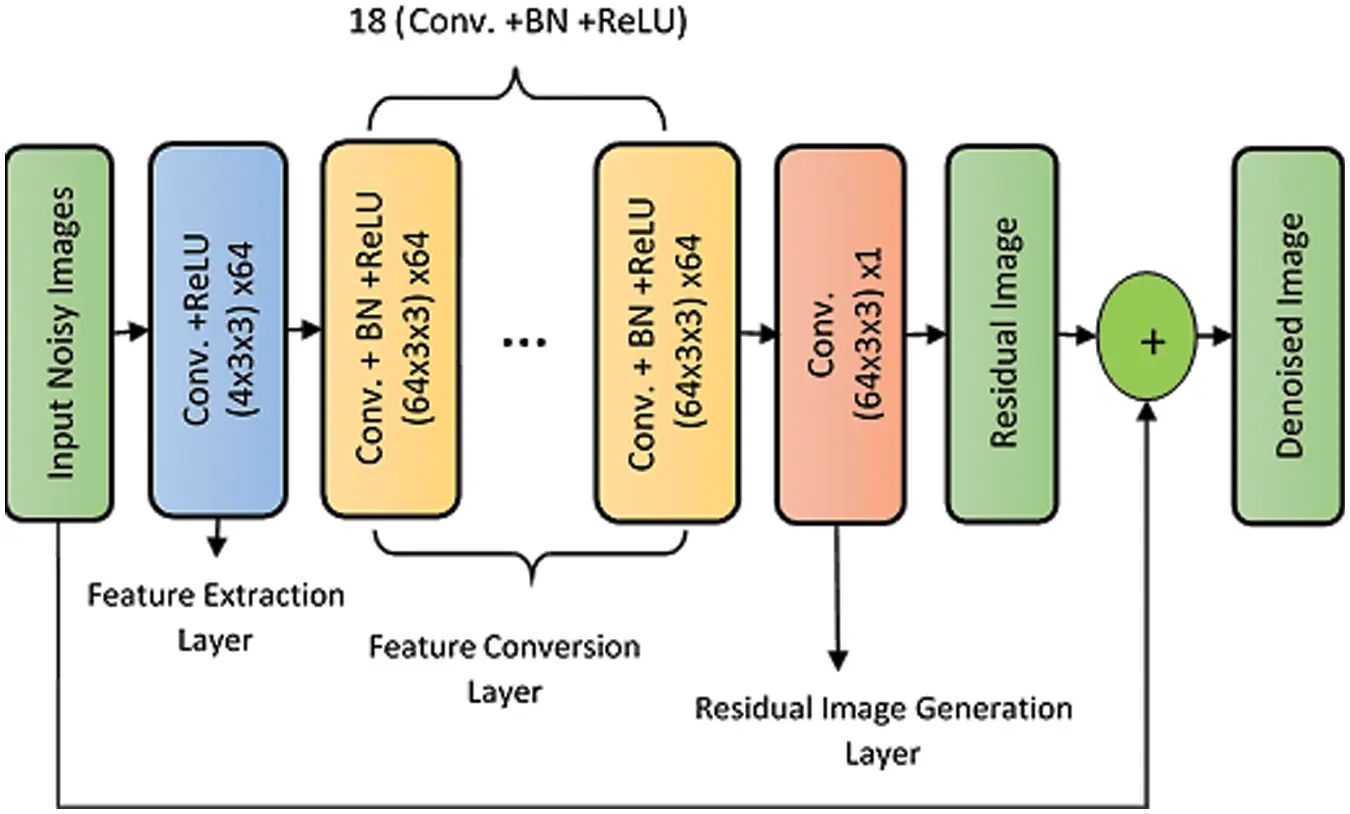Figure 2: The proposed CNN-based denoising model

Without deep residual learning, the input density and the convolutional feature are correlated with neighboring ones. Without BN, the problem of internal variable transformation aggravates.

4  Simulation Results and Discussions

Simulation results are presented using MATLAB R2019a on a Dell machine, Core i5 processor, 8 Gbytes RAMs, and 320 Gbytes hard disk. The metrics  used to measure the system performance are:

•   Peak Signal-to-Noise Ratio (PSNR) (dB)

PSNR=10log10maxI2MSE(17)

•   Correlation Coefficient (C r)

Cr=mn[(I(m,n)I¯)(In(m,n)I¯n)](mn(I(m,n)I¯)2)(mn(In(m,n)I¯n)2)(18)

The higher the PSNR and Cr values of the image, the better the image quality.

•   Processing Time

System complexity is expressed in terms of processing (CPU) time (seconds), which is calculated from the beginning of the simulation program to the end. The lower the CPU time of the image, the lower the algorithm complexity.

Five examples of scanned images were used. Also, different variances (0.2, 0.1, 0.05, 0.01) of speckle-noise are applied to simulate different scenarios. More than one type of filter has been applied to compare performance. Fig. 3 illustrates the performance of each algorithm applied to the Us image. The obtained PSNR and Cr values for each algorithm are calculated and tabulated for the Us image in Tab. 1. Gaussian noise was added to the other image modalities (X-ray, CT, PET, and MRI) with zero mean and different variances (0.01, 0.05, 0.1, and 0.2). To evaluate the subjective effect of these denoising methods, Figs. 4 to 7 show the performance of each algorithm applied on the X-ray, CT, PET, and MRI images, respectively. The obtained PSNR and Cr values for each algorithm are calculated and tabulated for each case in Tabs. 2 to 5, respectively.Figure 3: Obtained outcomes for the examined denoising filters for the US image at 0.05 speckle variance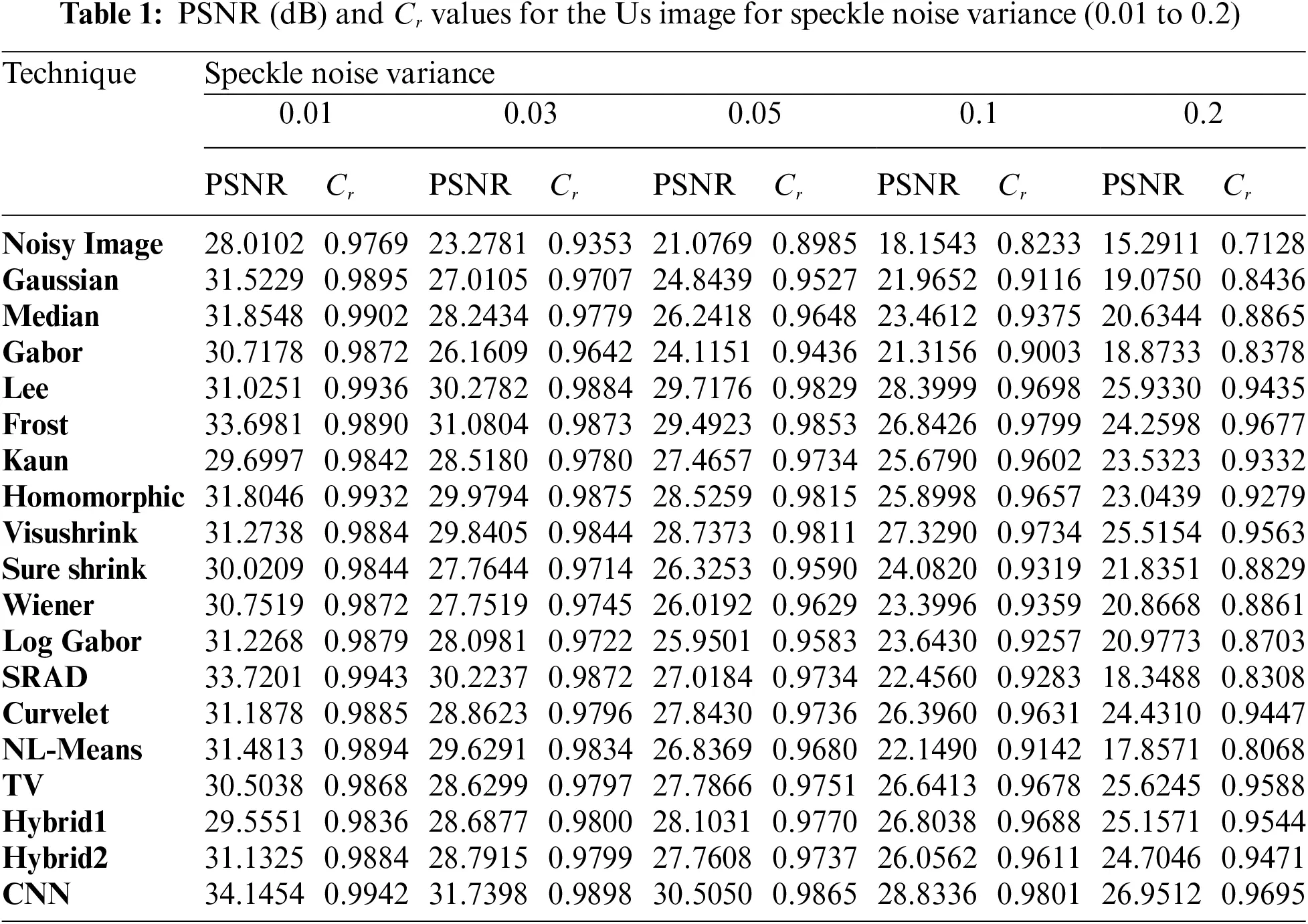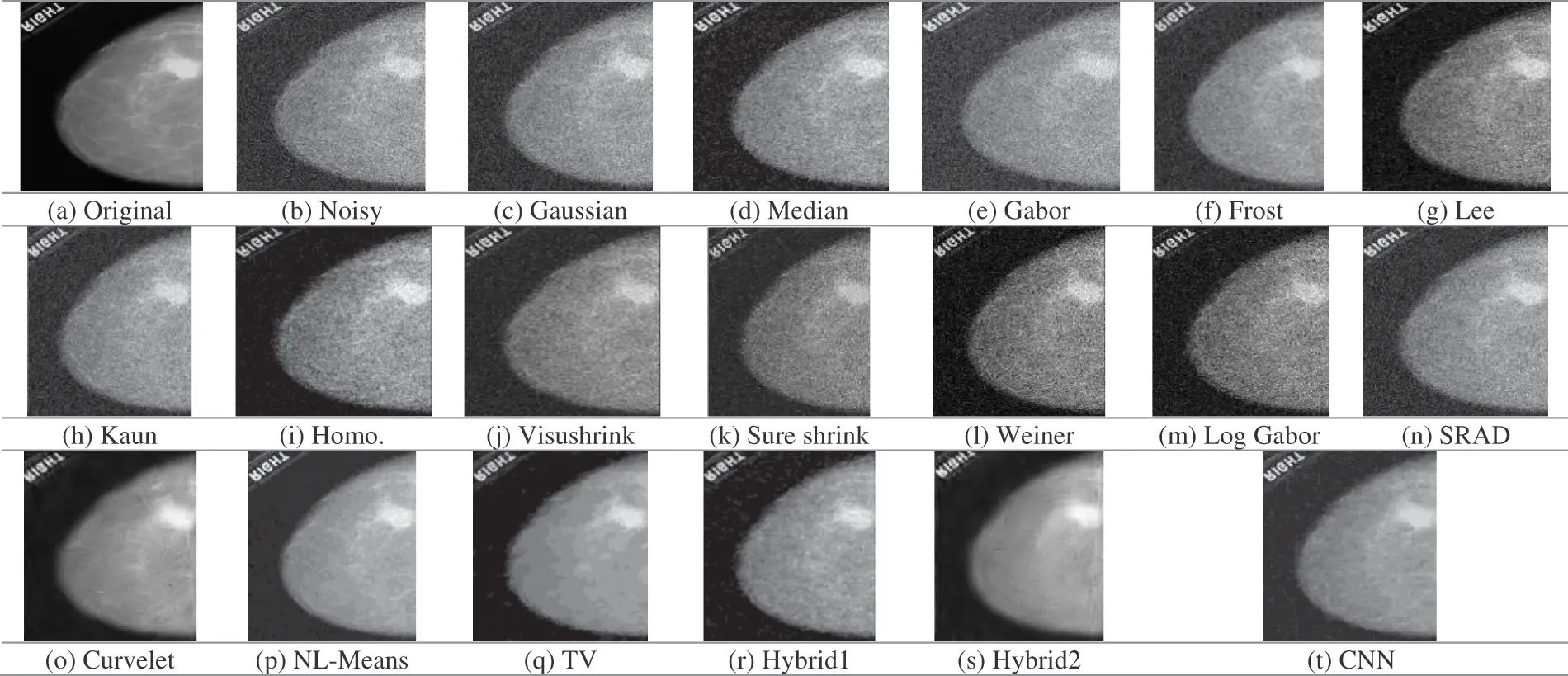Figure 4: Obtained outcomes for the examined denoising filters for the XR image at 0.05 gaussian variance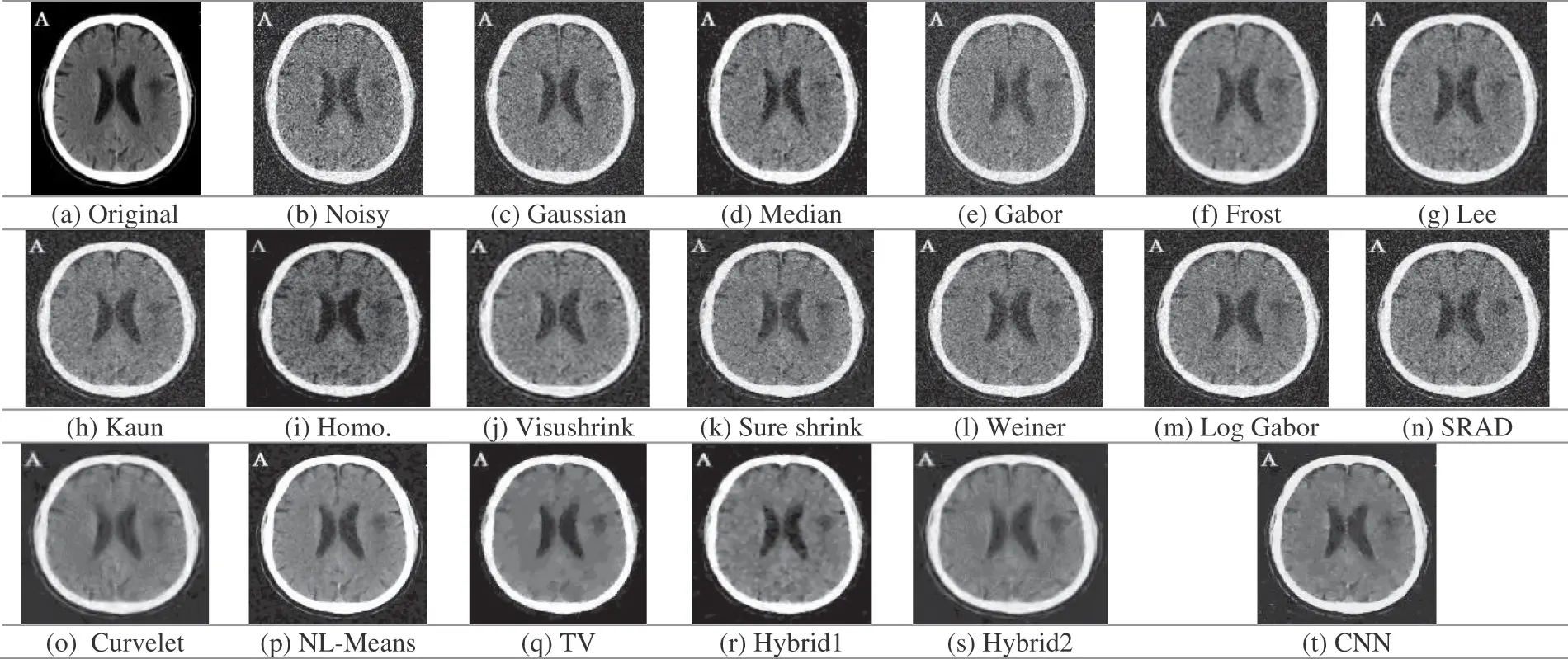Figure 5: Obtained outcomes for the examined denoising filters for the CT image at 0.05 gaussian variance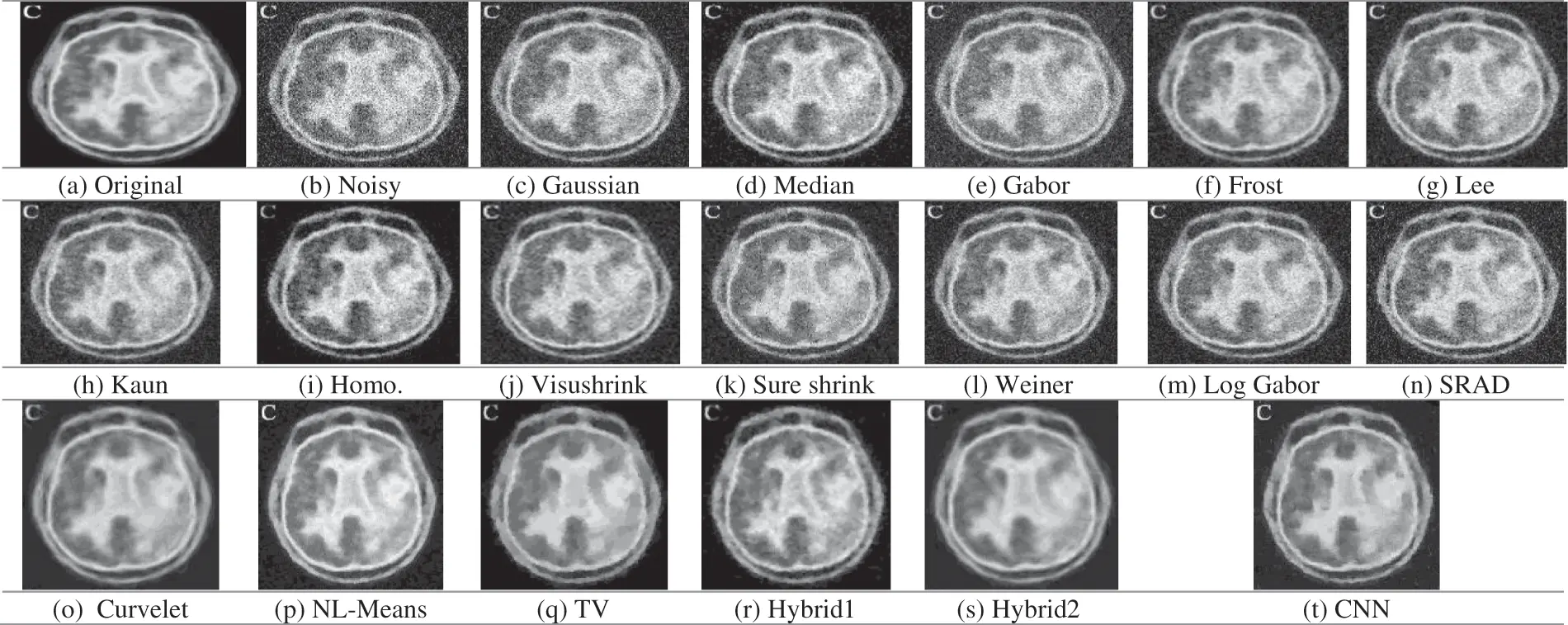Figure 6: Obtained outcomes for the examined denoising filters for the PET image at 0.05 gaussian variance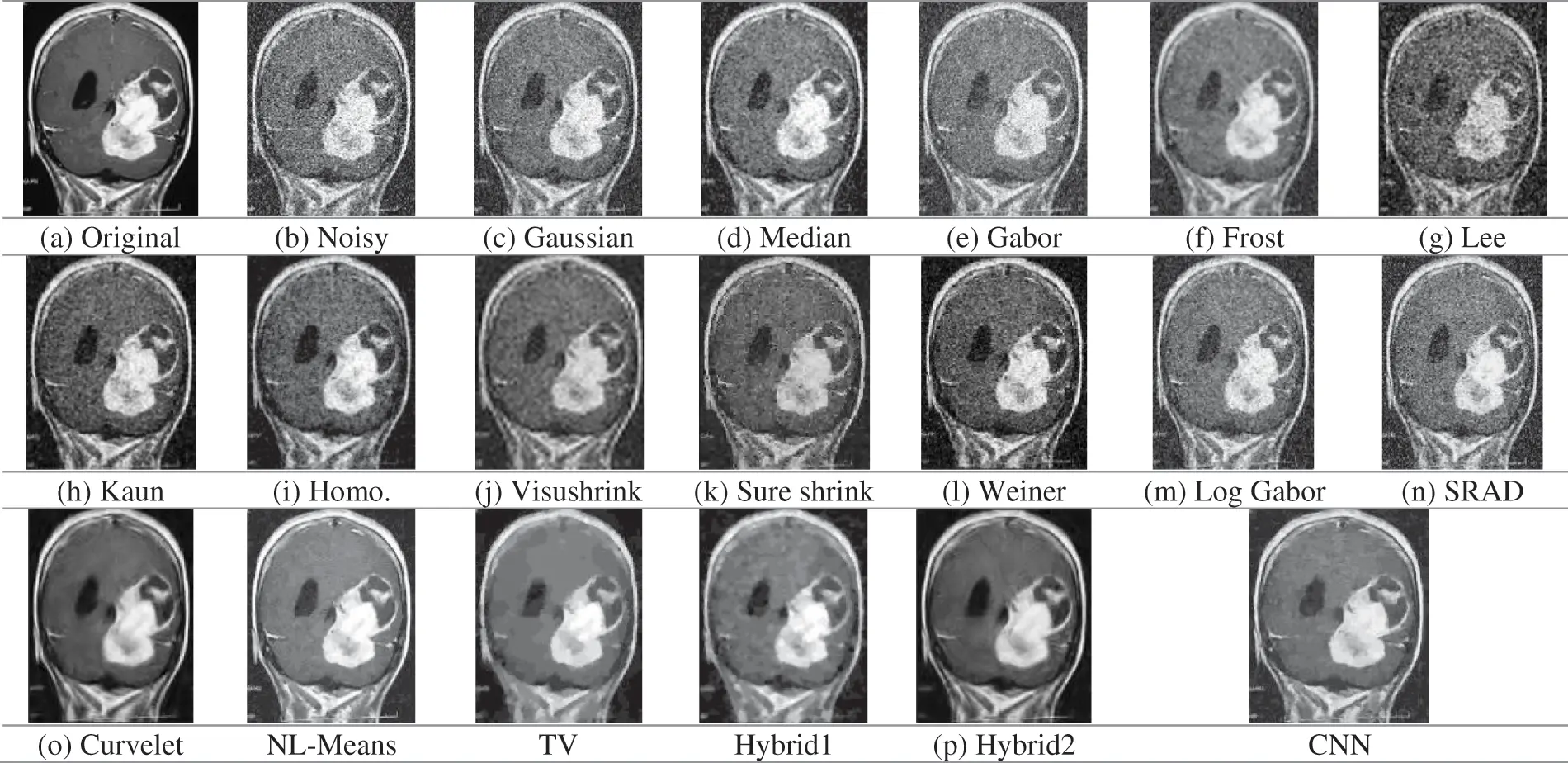Figure 7: Obtained outcomes for the examined denoising filters for the MRI image at 0.05 gaussian variance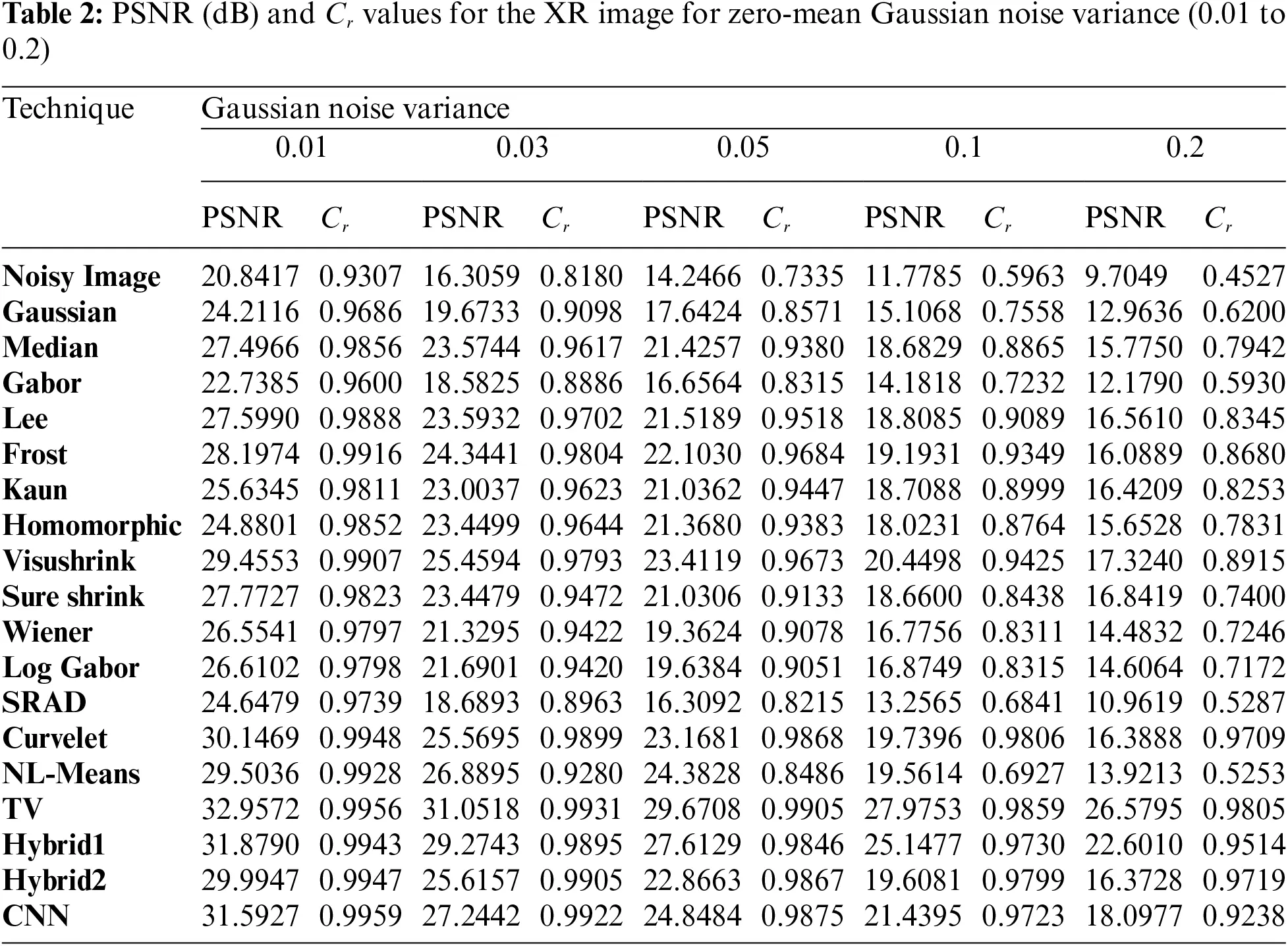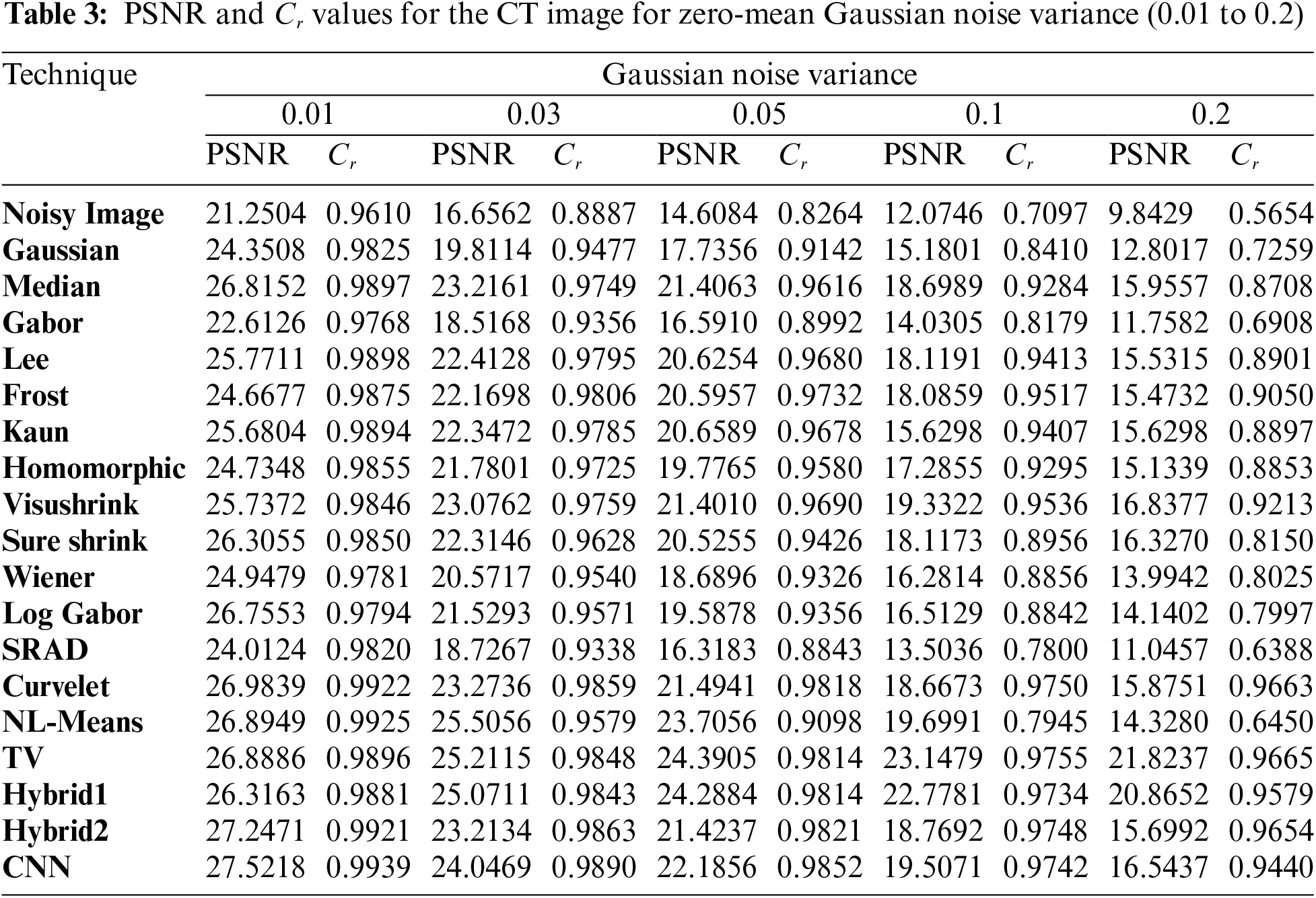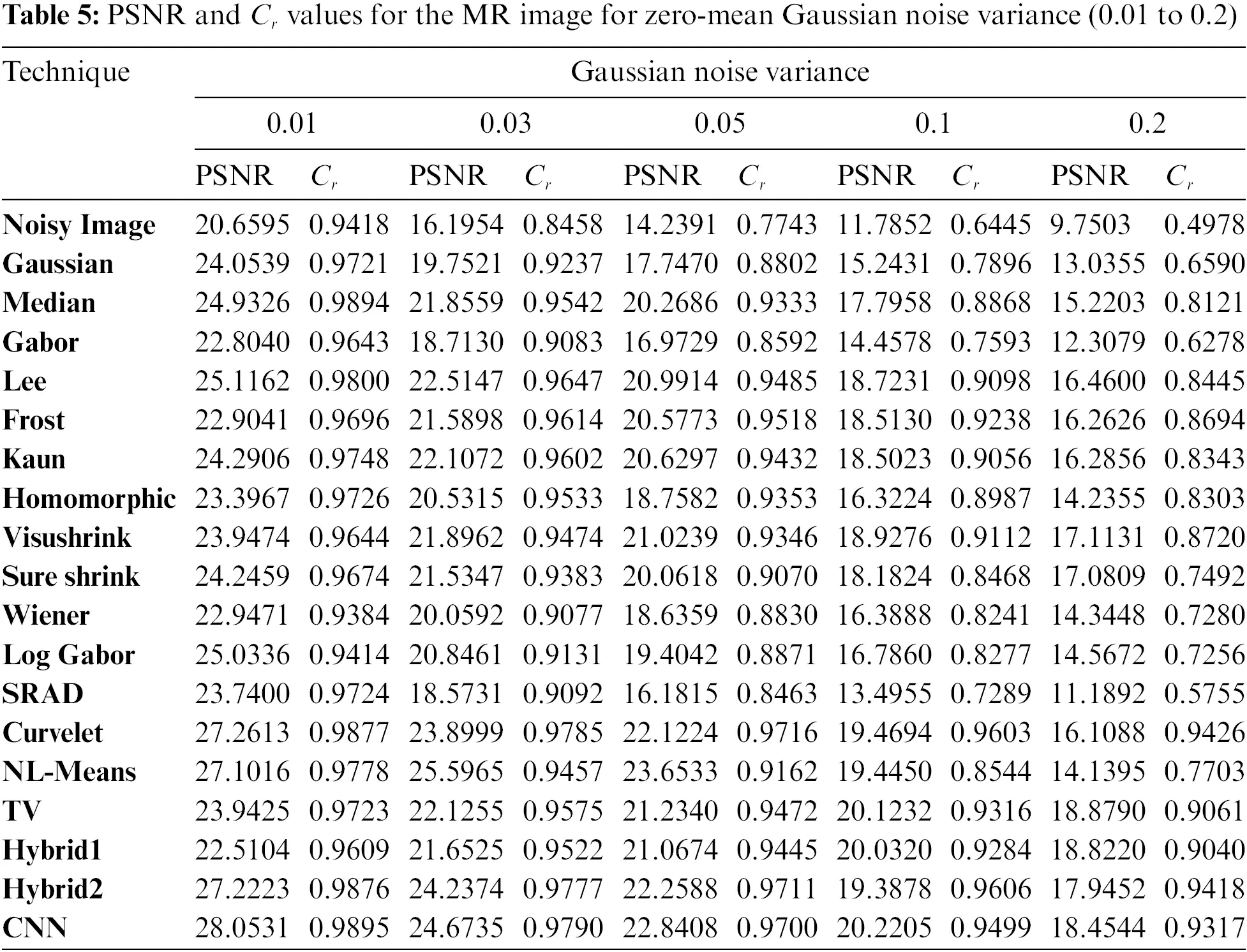Visual results of the Us breast image, shown in Fig. 3, reveal that the adaptive Lee, Kuan, and SRAD filters are superior to the other filtering techniques. They give the best image quality with preserved edges compared to Gaussian, Gabor, median, or log-Gabor. It is also apparent that the output of the denoising CNN outperforms most of the traditional denoising techniques. In addition, the visushrink and hybrid techniques have a smoothing effect.

From the presented results, the efficiency of the traditional filters is low when the noise level increases, as shown by the results of Cr and PSNR, respectively. On the contrary, the proposed CNN noise reduction model showed the best results at different speckle-noise levels, and it effectively reduces noise at the edges. The SRAD filter results in high results at lower noise levels on all the conventional filters. In Tab. 1, the suggested CNN model achieves the PSNR and Cr outcomes for all examined noise levels.

Fig. 4 depicts the visual results for the XR mammogram breast image. It is visible that most of the denoising techniques, including Gaussian, median, Gabor, Lee, Kuan, homomorphic, Wiener, Log-Gabor, and SRAD, failed to eliminate the Gaussian noise from the presented image. Visushrink, Curvelet, and hybrid two techniques decrease the resolutions of the image by more smoothing. The best results are obtained from the TV, hybrid2, NL-Means filter, and the denoising CNN model, but more details are visualized from NL-Means and the denoising CNN model.

Tab. 2 shows the PSNR and Cr results for XR images using different variance levels for Gaussian noise. TV, NL-Means, hybrid1, and CNN techniques show higher PSNR scores than other technologies. But TV technique gives the highest results in PSNR and Cr. However, the small details are missed in the output images. The NL-Means filter shows high image recovery efficiency by improving edge and texture. This clarifies that NL-Means and CNN models have more advantages on XR mammogram breast image.

Fig. 5 shows the original, noisy, and output denoising results of the CT brain images. The traditional denoising techniques, including Gaussian, median, Gabor, Lee, Kuan, homomorphic, wavelet-based, Log-Gabor, and SRAD techniques, poorly represented texture details, and poorly removed the Gaussian noise. On the other hand, the NL-Means filter and the denoising CNN model preserve edges and preserve texture details compared to the other techniques. Tab. 3 shows the Cr and PSNR values for noisy and denoised images. We have found that the TV and hybrid1 techniques have the best PSNR output values. Indeed, they have the highest value of PSNR of 24.3905 dB (for TV) and 24.2884 dB (for hybrid 1) compared to 23.7056 dB (for NL-Means) and 22.1856 dB (for the denoising CNN) at 0.05 Gaussian noise variance. Through the presented results, we conclude that the NL-Means and CNN models show the best results and are considered the best models in reducing noise and preserving accurate features in CT brain images.

Fig. 6 shows the outcomes of different noise reduction techniques of the PET brain images. According to the visualization, the Curvelet, hybrid2, TV, NL-Means, and CNN techniques eliminate the Gaussian noise efficiently. On the other hand, Gaussian, median, Gabor, Log-Gabor, and SRAD filters have a poor denoising performance. Tab. 4 demonstrates the PSNR and Cr values for Gaussian noise variance of 0.01, 0.03, 0.05, 0.1, and 0.2. Tab. 4 shows that the Curvelet, hybrid2, and CNN have the highest PSNR and Cr values at low noise variances. At high Gaussian noise variance, it is observed that the TV and hybrid1 techniques attain the best values for parameters PSNR and Cr. It appears from a perceptual and visual point of view that the Curvelet and the denoising CNN model restore edge and fine details more efficiently. Quantitatively, the TV and hybrid1 techniques have the best PSNR and Cr values.

Fig. 7 shows the original, noisy, and denoised output images of the MRI brain images. The MRI image, shown in Fig. 7a, has different regions, great details, smooth white, and dark regions with fine details. It is obviously shown in Fig. 7b that the noise severely degrades the image quality. Gaussian, median, Gabor, Lee, Weiner, Log-Gabor, and SRAD filters poorly deal with the image noise, and residual noise and the artifacts still exist. Wavelet-based techniques, visushrink, and sure shrink, smooth the image and affect the contrast heavily. Moreover, the Frost, Curvelet, and hybrid2 output results are blurred seriously. Fig. 7 shows the perceptual and visual superiority of the NL-Means and CNN models over the rest of the models.

The CNN model for Gaussian noise reduction excels from the MRI image in Tab. 5. The Cr values of hybrid2 and the CNN model are higher than hybrid1 and the TV techniques with lower Cr values. It is noticed that the CNN model is more efficient to denoise multimodal medical images with different noise types at low, medium, and high noise variances. As a result of using deep residual learning, the proposed model outperformed CNN by separating noise from noisy images.

Tab. 6 presents the CPU time for all the previously mentioned noise reduction techniques for the different image modalities. In the case of the Us image, models took much time to process because the Us image is the highest compared to the other image modalities due to the image size and the scan type. Due to the use of local statistics, the Lee filter outperforms adaptive filters based on wavelet and hybrid techniques in terms of processing speed. The Curvelet-based techniques have the highest processing time compared to the other techniques, up to 34 s for the Us image and 25 s for the other modalities. These techniques spend much more time because of the several transforms applied to the image before denoising. The denoising CNN model gives the most satisfying denoising results at a good CPU time, and it can also be considered an online processing model.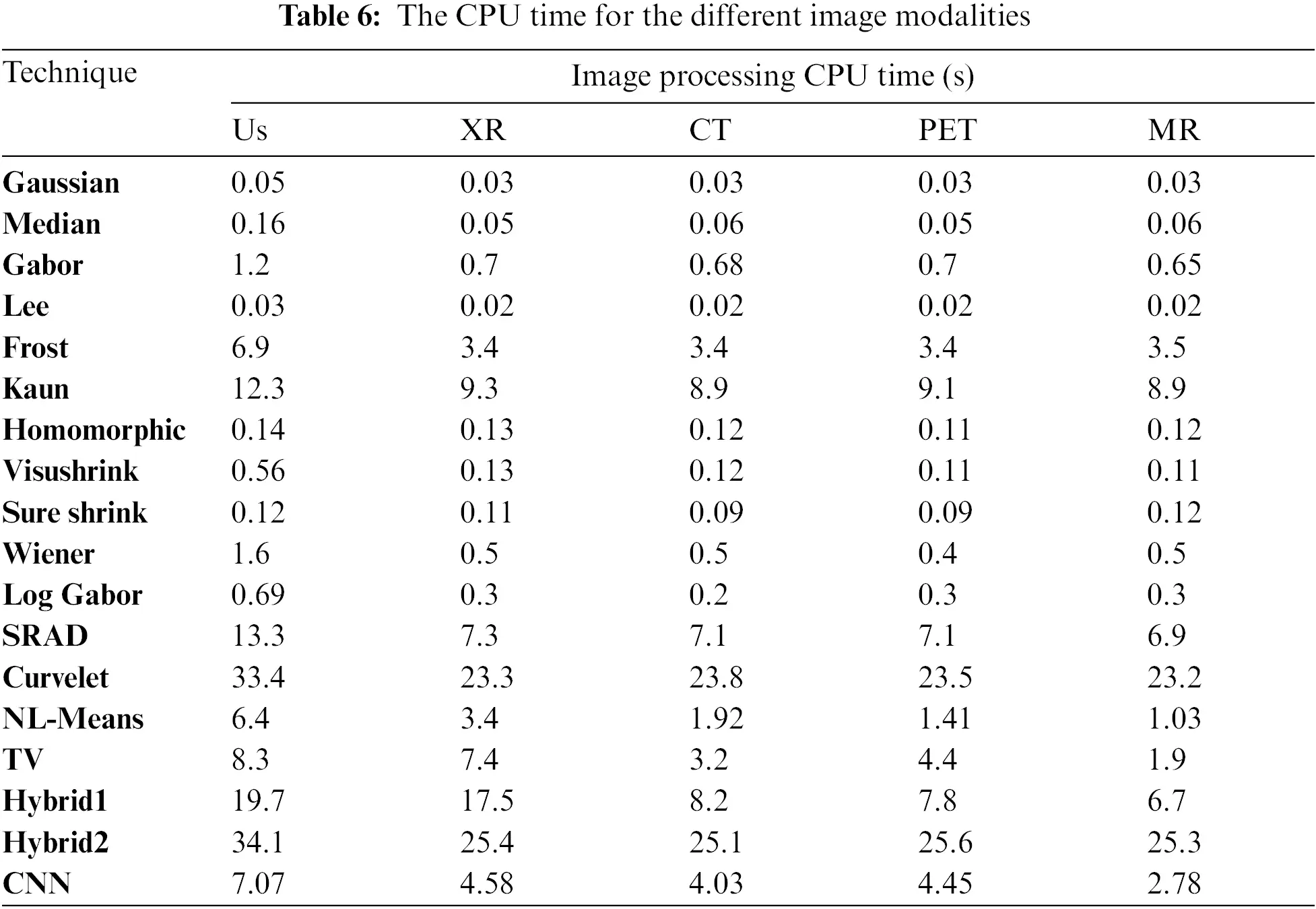•   Results Discussion

The obtained results demonstrate that the Gaussian and Gabor filters cannot remove Speckle or Gaussian noise, especially with large noise variances. The Gaussian filter also smoothes images and blurs the edges. The median filter is more robust than the Gaussian filter because it preserves edges. The Lee filter has a smoothing effect if the area has low variance. However, it fails to remove noise from areas closer to edges and lines. The Frost filter relies on adaptive filtering between pixels to reduce noise and smoothes the homogeneous regions. The Kuan filter has the advantage of preserving sharp edges compared to Frost and Lee filters. However, it is not effective at high noise variances.

The Homomorphic filter can maintain the brightness of images and increase the contrast. In the SRAD model, its performance decreases with increasing noise variance. However, it can remove speckle noise while maintaining edge features. In investigating various image denoising methods based on wavelet transform, it has been found that the sub-band adaptive thresholding methods outperformed the highest spatial domain method in the MRI image for all noise levels. If they did not perform better, they were slightly better than the Gaussian and Gabor filters. Out of the sub-band adaptive methods, sure-shrink consistently outperformed and visushrink. Because sure-shrink filtering is adaptive when dealing with images that contain abrupt changes or boundaries.

On the other hand, visushrink removes too many coefficients and overly smooths images. Wiener filter yields better results when the image is corrupted with a Gaussian noise rather than speckle noise. The Log-Gabor filter is poor when dealing with noisy images. The curvelet denoising technique is suitable when dealing with image edges and curves. The NL-mean filter is characterized by its ability to preserve the clarity of images and less information loss, especially at low noise variance. This is the result of taking the average of all pixels in the image as opposed to local mean filtering. The TV algorithms have advantages such as mean filtering and linear dimming that preserve edge characteristics and reduce noise in flat areas, at most high and low noise variances.

Hybrid algorithms are characterized by combining the features of discrete algorithms such as Hybrid Model 1, which outperforms other algorithms in terms of edges and structures. Hybrid Model 2 was also implemented by combining wavelet and curve transformation models. The curvelet transform model is characterized by representing curves in the images, and the wavelet transform model is characterized by reducing noise in smooth areas. One of the problems with the Hybrid Model 2 is that it takes a longer time to process. However, it significantly outperforms wavelet-based technologies.

In contrast to traditional noise reduction techniques, the CNN noise reduction model can handle noisy images with different noise levels. It also reduces quantitative and qualitative noise. The CNN model is characterized by its ability to adapt in all modalities of medical images, unlike other models that excel in some modalities and fail in other modalities of images. Moreover, visual comparisons of the different algorithms show that the CNN noise reduction model produces more perceptive images with sharp edges and finer information.

5  Conclusions and Future Work

This paper presented a CNN-based denoising model and a comparative study of noise reduction methods for various medical image modalities. The performance of all employed methods is tested on different medical images (Us, X-ray, CT, PET, and MR). This study can summarize all the noticed advantages and disadvantages of the tested noise reduction techniques employed in this paper on different medical image modalities. The employed algorithms are tested on the medical images, and their denoising performance has been compared and studied. For the spatial domain, median filtering outperformed the Gaussian filtering. The superiority of the waveform model in reducing Gaussian noise was proven to be significantly superior to the rest of the other models. It has shown the best image quality. From comparing the denoising results of different denoising techniques using different threshold functions, it is obvious that the images become blurred after global threshold denoising. On the other hand, the image texture details are well preserved using the wavelet denoising method based on adaptive thresholding. The comparison is more obvious in the MRI image, which has more texture details. Similar results can be obtained for other test images. The curvelet algorithms excel at reducing noise in some image modalities such as curvilinear images and linear singularities. Moreover, the hybrid algorithm based on combining wavelet and curvelet transforms maximizes the advantages of both. The numerical and visual results show that the proposed CNN noise reduction model is adapted to all modalities of medical images in terms of perceptual and visual quality. It also possesses high scores in most noise levels and image modalities. Therefore, we conclude that the CNN model is superior to traditional filtering and noise reduction techniques. The CNN model also has a good denoising CPU time. For future work, combinations of other transforms and the adaptation of CNN models could yield better results than those obtained separately.

Acknowledgement: Princess Nourah bint Abdulrahman University Researchers Supporting Project number (PNURSP2022R66), Princess Nourah bint Abdulrahman University, Riyadh, Saudi Arabia.

Funding Statement: Princess Nourah bint Abdulrahman University Researchers Supporting Project number (PNURSP2022R66), Princess Nourah bint Abdulrahman University, Riyadh, Saudi Arabia.

Conflicts of Interest: The authors declare that they have no conflicts of interest to report regarding the present study.

## References

1. W. El-Shafai, “Joint adaptive pre-processing resilience and post-processing concealment schemes for 3D video transmission,” 3D Research, vol. 6, no. 1, pp. 1–21, 2015.
2. W. El-Shafai, S. El-Rabaie, M. El-Halawany and F. Abd El-Samie, “Encoder-independent decoder-dependent depth-assisted error concealment algorithm for wireless 3D video communication,” Multimedia Tools and Applications, vol. 77, no. 11, pp. 13145–13172, 2018.
3. M. Diwakar and M. Kumar, “A review on CT image noise and its denoising,” Biomedical Signal Processing Control, vol. 42, no. 5, pp. 73–88, 2018.
4. W. El-Shafai, S. El-Rabaie, M. El-Halawany and F. Abd El-Samie, “Enhancement of wireless 3D video communication using color-plus-depth error restoration algorithms and Bayesian Kalman filtering,” Wireless Personal Communications, vol. 97, no. 1, pp. 245–268, 2017.
5. W. El-Shafai, S. El-Rabaie, M. El-Halawany and F. Abd El-Samie, “Recursive Bayesian filtering-based error concealment scheme for 3D video communication over severely lossy wireless channels,” Circuits Systems, and Signal Processing, vol. 37, no. 11, pp. 4810–4841, 2018.
6. H. El-Hoseny, W. El-Rahman, W. El-Shafai, S. M. El-Rabaie, K. Mahmoud et al., “Optimal multi-scale geometric fusion based on non-subsampled contourlet transform and modified central force optimization,” International Journal of Imaging Systems and Technology, vol. 29, no. 1, pp. 4–18, 2019.
7. B. Goyal, A. Dogra, S. Agrawal and B. Sohi, “Noise issues prevailing in various types of medical images,” Biomedical & Pharmacology Journal, vol. 11, no. 3, pp. 1227–1237, 2018.
8. L. Fan, F. Zhang and H. Fan, “Brief review of image denoising techniques,” Visual Computing for Industry, Biomedicine, and Art, vol. 2, no. 7, pp. 1–12, 2019.
9. B. Goyal, A. Dogra, S. Agrawal, B. Sohi and A. Sharma, “Image denoising review: From classical to state-of-the-art modeles,” Information Fusion, vol. 55, no. 7, pp. 220–244, 2020.
10. S. Garg, R. Vijay and S. Urooj, “Statistical model to compare image denoising techniques in medical MR images,” Procedia Computer Science, vol. 152, no. 3, pp. 367–374, 2019.
11. A. Sedik, H. Emara, A. Hamad, E. Shahin, N. El-Hag et al., “Efficient anomaly detection from medical signals and images,” International Journal of Speech Technology, vol. 22, no. 3, pp. 739–767, 2019.
12. S. Singh and I. Singula, “Enhanced face matching technique-based Gabor Filter and PSO,” International Journal of Advanced Research in Computer Science and Software Engineering, vol. 4, no. 8, pp. 1215–1220, 2014.
13. W. El-Shafai, S. El-Rabaie, M. El-Halawany and F. Abd El-Samie, “Proposed dynamic error control techniques for QoS improvement of wireless 3D video transmission,” International Journal of Communication Systems, vol. 31, no. 10, pp. 1–23, 2018.
14. W. El-Shafai, S. El-Rabaie, M. El-Halawany and F. Abd El-Samie, “Proposed adaptive joint error-resilience concealment algorithms for efficient colour-plus-depth 3D video transmission,” IET Image Processing, vol. 12, no. 6, pp. 967–984, 2018.
15. A. Chaari, K. Kammoun, I. Kallel, M. Frikha and J. Feki, “Performance evaluation of various denoising filters and segmentation methods for OCT images,” in Proc. 5th Int. Conf. on Advanced Technologies for Signal and Image Processing (ATSIP), Sousse, Tunisia, pp. 1–6, 2020.
16. B. Ahamed, D. Yuvaraj and S. Priya, “Image denoising with linear and non-linear filters,” in Proc. Int. Conf. on Computational Intelligence and Knowledge Economy (ICCIKE), Dubai, United Arab Emirates, pp. 806–810, 2019.
17. W. El-Shafai, S. El-Rabaie, M. El-Halawany and F. Abd El-Samie, “Performance evaluation of enhanced error correction algorithms for efficient wireless 3D video communication systems,” International Journal of Communication Systems, vol. 31, no. 1, pp. 1–27, 2018.
18. A. Odat, M. Otair and F. Shehadeh, “Image denoising by comprehensive median filter,” International Journal of Applied Engineering Research, vol. 10, no. 15, pp. 36016–36022, 2015.
19. S. Orcioni, A. Paffi, F. Camera, F. Apollonio and M. Liberti, “Automatic decoding of input sinusoidal signal in a neuron model: High pass homomorphic filtering,” Neurocomputing, vol. 292, no. 2, pp. 165–173, 2018.
20. H. El-Hoseny, W. Abd El-Rahman, W. El-Shafai, G. El-Banby, E. El-Rabaie et al., “Efficient multi-scale non-sub-sampled shearlet fusion system based on modified central force optimization and contrast enhancement,” Infrared Physics & Technology, vol. 10, no. 2, pp. 102–123, 2019.
21. H. Hammam, W. El-Shafai, E. Hassan, A. El-Azm, M. Dessouky et al., “Blind signal separation with noise reduction for efficient speaker identification,” International Journal of Speech Technology, vol. 4, no. 6, pp. 1–16, 20
22. N. El-Hag, A. Sedik, W. El-Shafai, H. El-Hoseny, A. Khalaf et al., “Classification of retinal images based on convolutional neural network,” Microscopy Research and Technique, vol. 84, no. 3, pp. 394–414, 2021.
23. A. Mahmoud, W. El-Shafai, T. Taha, E. El-Rabaie, O. Zahran et al., “A statistical framework for breast tumor classification from ultrasonic images,” Multimedia Tools and Applications, vol. 80, no. 4, pp. 5977–5996, 2021.
24. W. El-Shafai, E. El-Rabaie, M. Elhalawany and F. Abd El-Samie, “Improved joint algorithms for reliable wireless transmission of 3D color-plus-depth multi-view video,” Multimedia Tools and Applications, vol. 78, no. 8, pp. 9845–9875, 2019.
25. Y. Zhan, W. Ding and F. Pan, “Improved wavelet threshold for image de-noising,” Frontiers in Neuroscience, vol. 13, no. 1, pp. 1–39, 2019.
26. V. Strela, “Denoising via block wiener filtering in wavelet domain,” in Proc. IEEE 3rd European Congress of Mathematics, Barcelona, Birkhäuser Verlag, pp. 619–625, 2001.
27. W. El-Shafai, S. El-Rabaie, M. El-Halawany and F. Abd El-Samie, “Efficient hybrid watermarking schemes for robust and secure 3D-MVC communication,” International Journal of Communication Systems, vol. 31, no. 4, pp. 1–22, 2018.
28. K. Abdelwahab, S. Abd El-atty, W. El-Shafai, S. El-Rabaie and F. Abd El-Samie, “Efficient SVD-based audio watermarking technique in FRT domain,” Multimedia Tools and Applications, vol. 79, no. 9, pp. 5617–5648, 2020.
29. J. Rajan, K. Kannan and M. Kaimal, “An improved hybrid model for molecular image denoising,” Journal of Mathematical Imaging and Vision, vol. 31, no. 3, pp. 73–79, 2008.
30. A. Mahmoud, S. Rabaie, T. Taha and F. Abd El-Samie, “Mixed curvelet and wavelet transforms for speckle noise reduction in ultrasonic b-mode images,” Information Sciences and Computing, vol. 3, no. 9, pp. 1–21, 2015.
31. O. Faragallah, A. Afifi, W. El-Shafai, H. El-Sayed, E. Naeem et al., “Investigation of chaotic image encryption in spatial and FrFT domains for cybersecurity applications,” IEEE Access, vol. 8, pp. 42491–42503, 2020.
32. K. Zhang, W. Zuo, Y. Chen, D. Meng and L. Zhang, “Beyond a gaussian denoiser: Residual learning of deep CNN for image denoising,” IEEE Transactions on Image Processing, vol. 26, no. 7, pp. 3142–3155, 2017.This work is licensed under a Creative Commons Attribution 4.0 International License, which permits unrestricted use, distribution, and reproduction in any medium, provided the original work is properly cited.